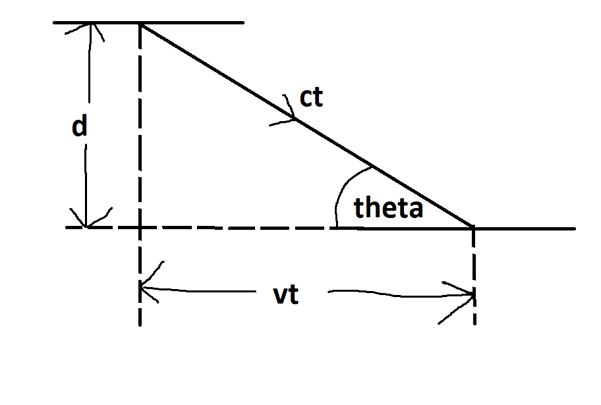# Derivation of special relativity formulae

I was bored, so I decided to derive the special relativity formulae.
I drew the following diagram of a light clock:In order to find t, I did sinθ=d/ct
Which gives tsinθ=d/c
Which gives t=d/csinθ

If v = 0, vt = 0, and θ = 90
sin90 = 1
t = d/csinθ = d/c
We call this t0

If v is greater than 0, vt is greater than zero, and θ is less than 90
sin90 is less than1
t = d/csinθ is greater than d/c

We use Pythagoras to get t0=t(1- (v2/c2))-1/2

What are the steps involved in getting to this answer?

#### Attachments

Dale
Mentor
2021 Award
In order to find t, I did sinθ=d/ct
Usually both t and θ are considered unknowns. So writing one equation in two unknowns doesn't help. You should use the Pythagorean theorem instead to get one equation in one unknown.

•Clever Penguin
Usually both t and θ are considered unknowns. So writing one equation in two unknowns doesn't help. You should use the Pythagorean theorem instead to get one equation in one unknown.

Valid pointso (ct)2 = d2 + (vt)2

Last edited:
Dale
Mentor
2021 Award
Valid pointso (ct)2 = d2 + (vt)2
Yes. Then rearranging and using ##d/c=t_0## gives you the desired formula

Yes. Then rearranging and using ##d/c=t_0## gives you the desired formula

thanks